# Canvas: Using Python to create Calculated Questions

## Background

I just recently started with Canvas because Uppsala University has decided to use it as its upcoming LMS platform after a failed attempt with another product. Therefore I had already spent some time with Blackboard and was quite fond of the calculated questions type in quizzes. I quickly found out that Canvas offers essentially the same functionality but a bit less comfortable.

## Problem

A calculated question or Formula Question as it is called in the interface of Canvas is based on a table of pre-generated variable values and corresponding results. In the general case the variables are defined and the target function is entered using the web interface, then Canvas calculates random number values for the variables and the resulting answer value. However, as the designer you have no possibility to influence the variable values afterwards (unlike in Blackboard where you have a spreadsheet-like interface). Also, in Canvas, the equation cannot be altered once it has been entered – and the supported syntax is not very convenient for more complex problems.
I was also missing the ability to give a relative tolerance for the correct answers in a question, however, I found out that entering a percentage-sign exactly gives this behavior even though it does not seem documented anywhere.

## Solution or problems?

My hope was then for the API, since it seemed to support the creation of questions. But even though there is a Python library for the purpose of controlling Canvas, many of the functions are not very well documented. My first tries failed miserably but finally I was on the right track.

The cause of my problems was that the Canvas API uses different field identifiers and structures when creating a calculated question as when you retrieve the contents of an already existing question, as I of course did in my attempts to reverse-engineer the interface.

## Working solution

Here is now an example for a working solution to give you full control over the generation of Formula Qeustions using Python and the canvasapi library. The example is in Python 3 and creates a question from the field of electronics – the voltage in a voltage divider. The Python script generates the variables, fills the variables with random numbers from a set of predefined, commonly used values. I tried to write the script more for readability than any pythonic optimization.

Since this is mostly the result of successful reverse engineering and not based on the actual source code of Canvas the above example should perhaps be used with care, but for me it is what I needed to create usable questions for my students. Perhaps this could also serve the developers as an example on how the interface for calculated questions could be improved in the future.

## How does it work?

The dictionary variables (lines 26-49) contains the names and ranges of the variables, as well as formatting instructions. The ranges are given as lists. In lines 61-66 the random values are generated and the results calculated from these values. Lines 70-77 create a rudimentary table to be included in the question text containing the variables and their values as well as physical units for this particular question. Lines 80-93 finally assemble the variable/answer block and lines 109-128 put everything together into the dictionary to create a new question.

The script then inserts the question into an existing quiz in an existing course in line 140.

### After running the script

This screenshot shows the inserted question after running the script, obviously this would need some more cosmetics.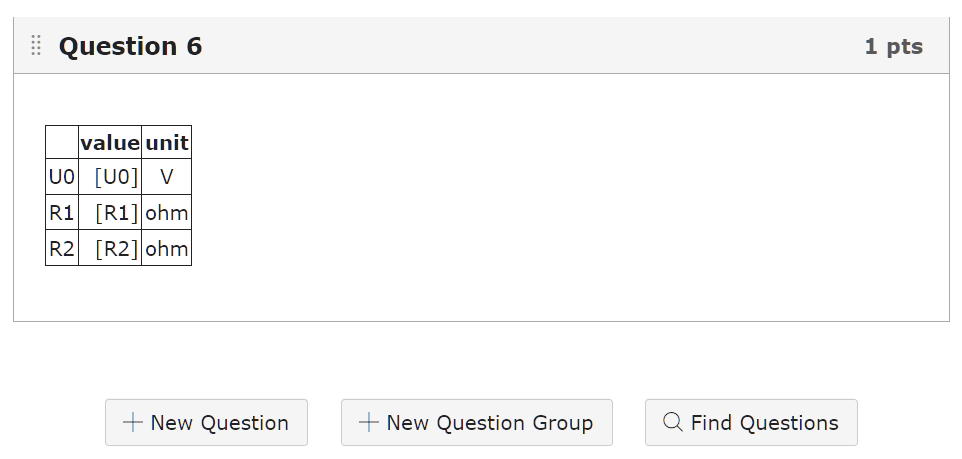And when editing the question this is what you see: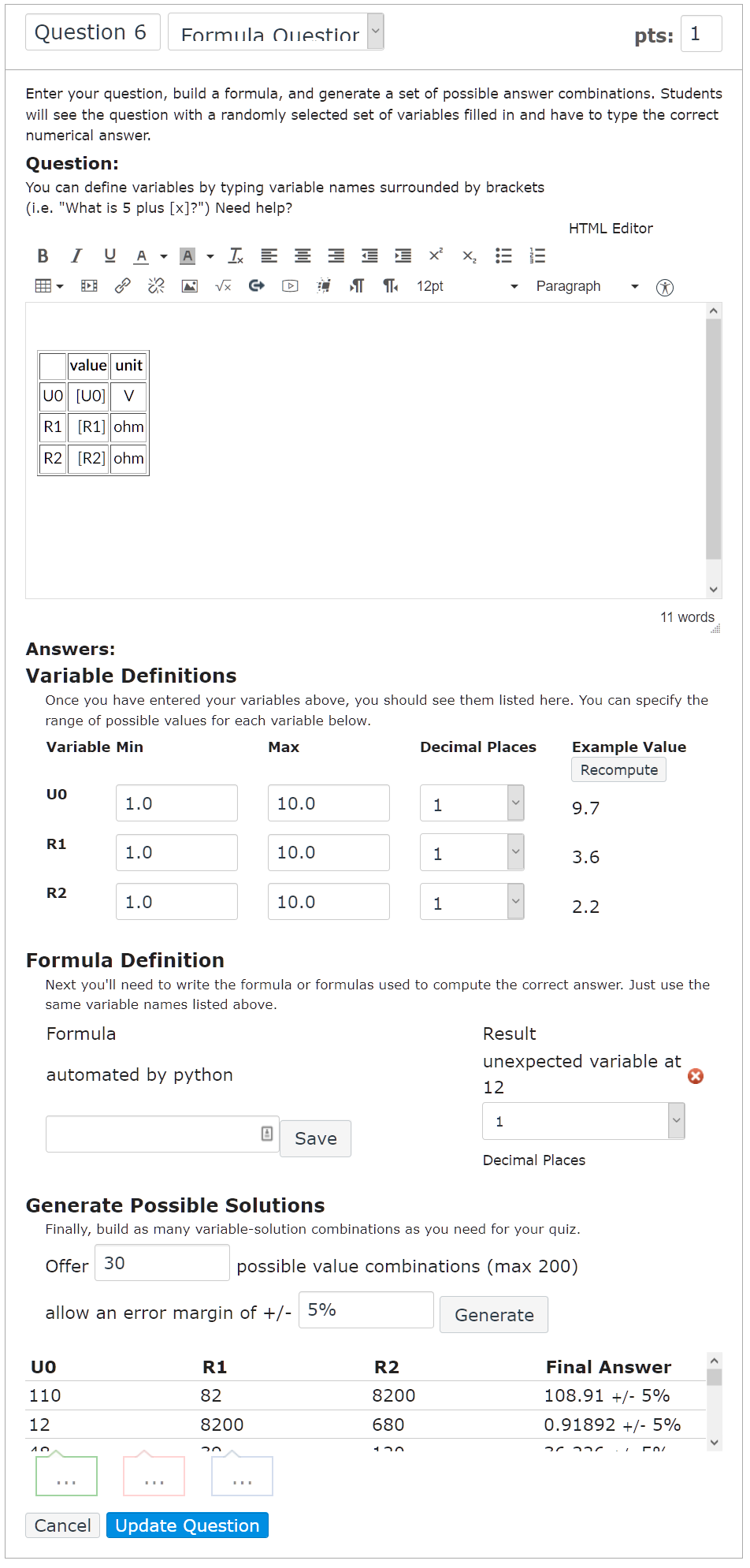Be careful not to touch the variables or the formula section since this will reset the table values.

### Cosmetics

In order to be presentable to the students the above questions needs some cosmetics. What is to be calculated? Perhaps insert a picture or an equation? More text?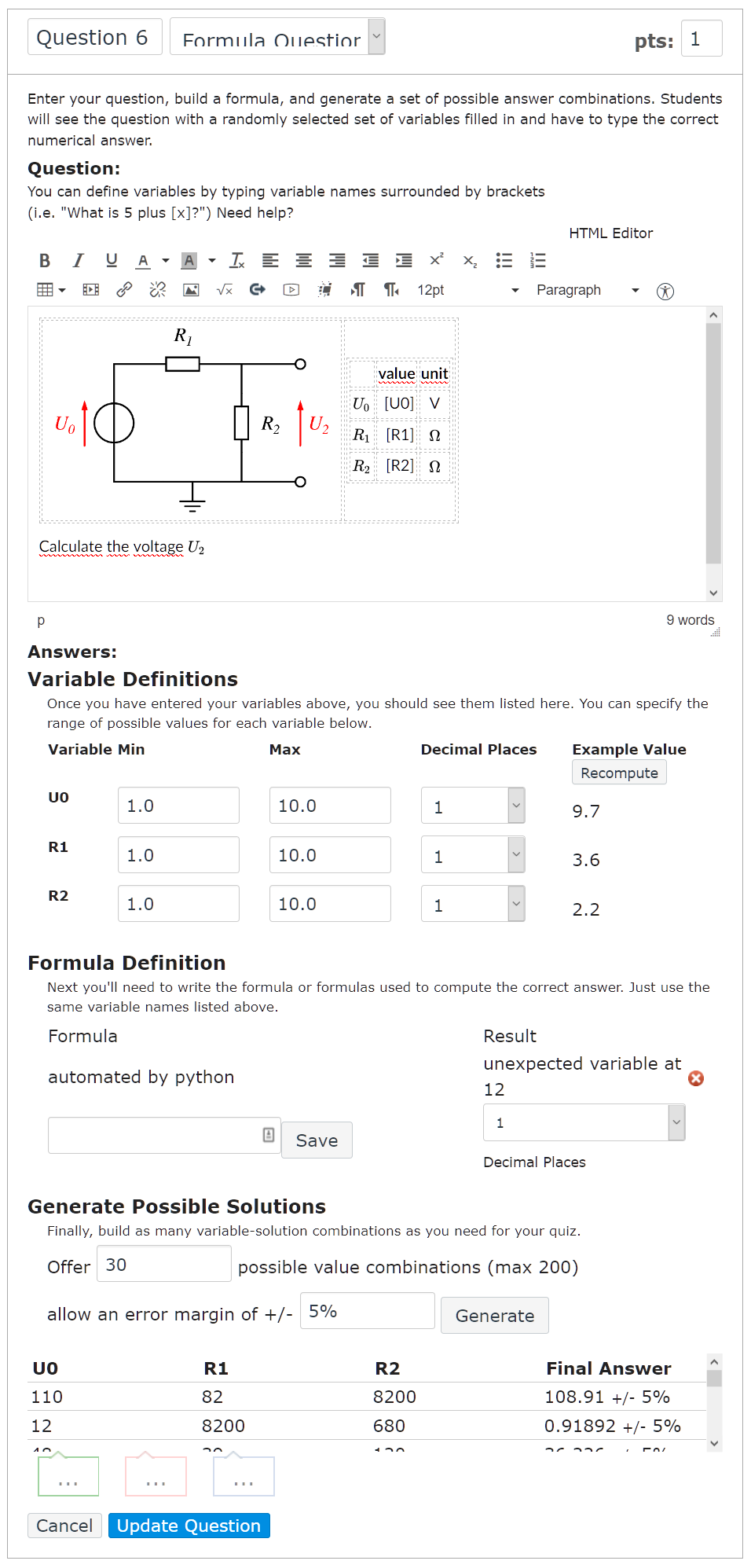After updating the question and leaving the editor it now looks like this in the Canvas UI: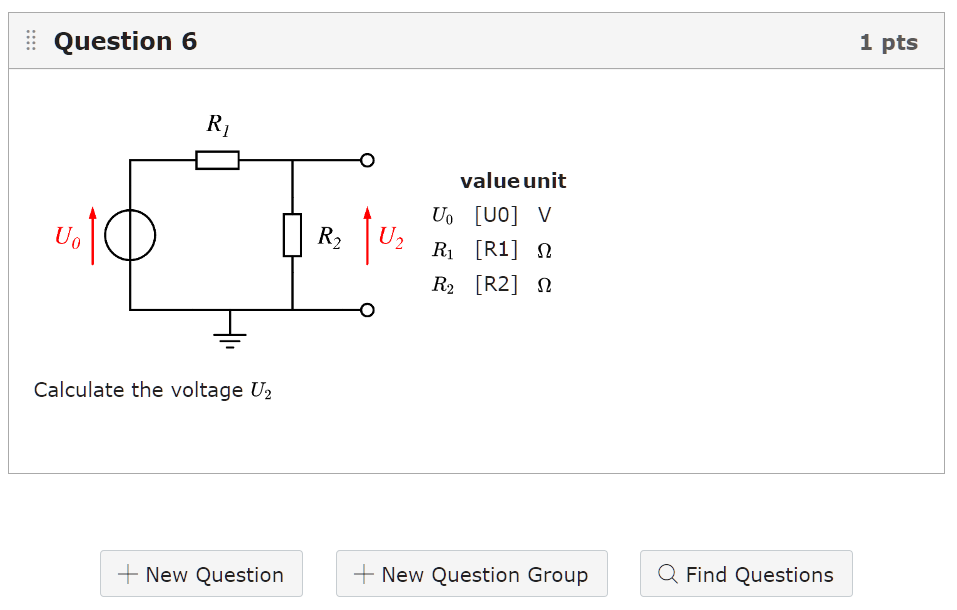### Seeing and answering the question

When you now start the quiz, this is how the question looks: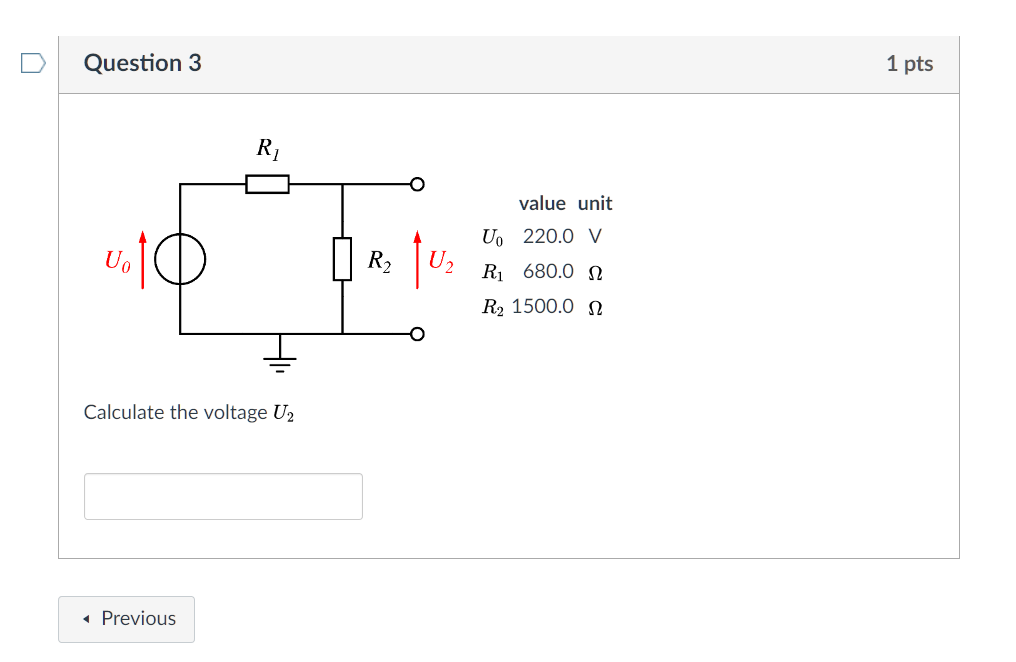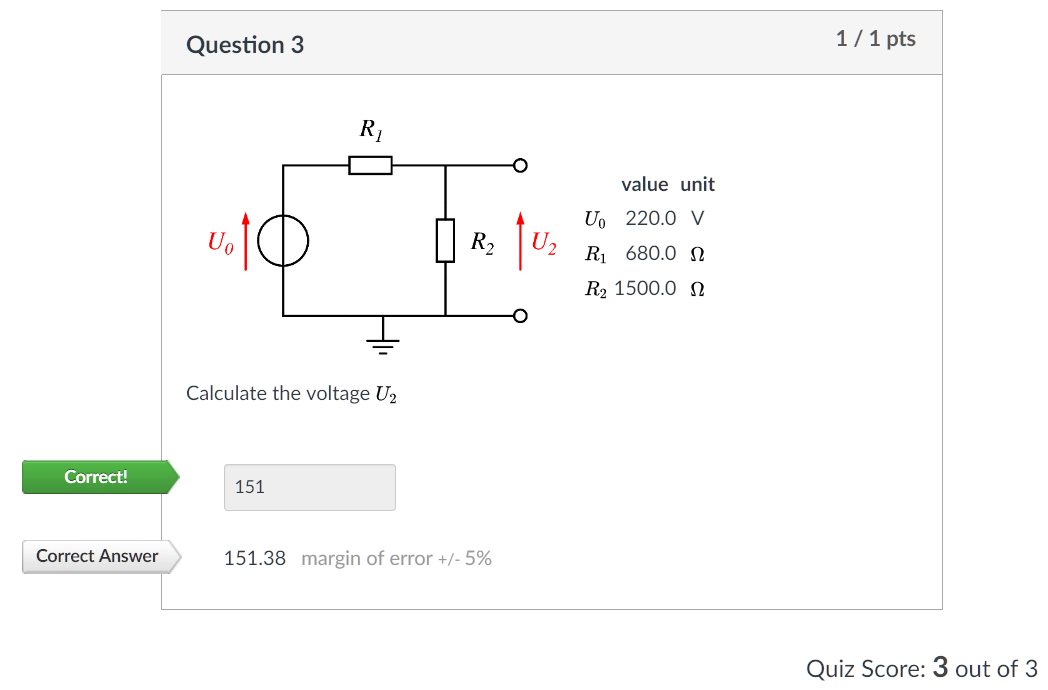## Summary

• calculated_questions can be generated using the Python canvasapi library
```'answers': [
{
'weight': '100',
'variables': [
{'name': 'U0', 'value': '9.0'},
{'name': 'R1', 'value': '5600.0'},
{'name': 'R2', 'value': '5600.0'}],
```answers=[
` 'formulas':  ['a*b'],‍‍`
` formulas=[{'formula': 'a*b'}],‍‍`